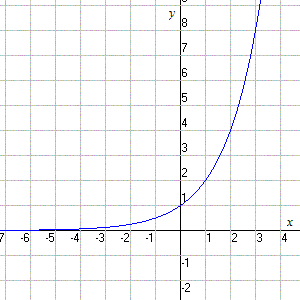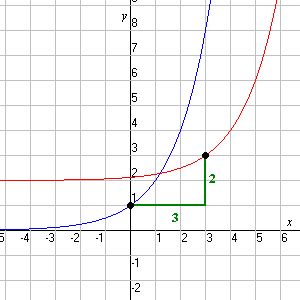# Exponential Functions

The "basic" exponential function is the function

$y={a}^{x}$

where $a$ is some positive constant.

For example, the graph of $y={2}^{x}$ looks like this:Note that:

$1$ ) The $y$ -intercept is $1$ (no matter what the value of $a$ is).

$2$ ) The graph approaches the $x$ -axis asymptotically as $x$ goes to negative infinity (or as $x$ goes to positive infinity, if $0 ).

$3$ ) The graph is always positive (never zero or negative).

The exponential function can be shifted $k$ units upwards and $h$ units to the right with the equation:

Example:

Graph the equation.

Start with the "basic" exponential graph $y={2}^{x}$ . Then shift the graph three units to the right and two units up.